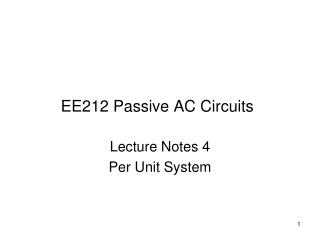DownloadDownload PresentationEE212 Passive AC Circuits

# EE212 Passive AC Circuits

Télécharger la présentation## EE212 Passive AC Circuits

- - - - - - - - - - - - - - - - - - - - - - - - - - - E N D - - - - - - - - - - - - - - - - - - - - - - - - - - -
##### Presentation Transcript

1. EE212 Passive AC Circuits Lecture Notes 4 Per Unit System

2. Per Unit (p.u.) System Computations related to power systems involving machines and transformers are often carried out in p.u. system. In p.u. system, power system quantities (such as V, I, R, X, VA, P and Q) are expressed as decimal fractions of chosen base values. p.u values are sometimes expressed in percentage (beween 0 to 100%). Any value can be taken as the Base Value. However, it is chosen to make computation easy. It is usually taken equal to the rating of a machine/transformer in the power circuit. Power equipment impedance ratings are usually given in p.u. (expressed in %) based on their rated voltage and VA.

3. Advantages of the p.u. System p.u. values of circuit parameters are the same on either side a transformer. We don’t have to worry about circuit parameters being referred to one side or the other. Machines and transformer constants (e.g. resistance, leakage/transient/sub-transient reactance, excitation current, etc.) lie within a narrow range of values when expressed in p.u. We can therefore guess reasonable values for approximate calculations, or easily estimate their correctness. e.g. Leakage reactance of autotransformers lie within 0.01 – 0.04 p.u. (or 1% - 4%).

4. Applying the p.u. Method • Choose base values for 2 quantities, VA and V. Other base quantities (i.e. Ibase and Zbase) are determined from the chosen VAbase and Vbase. • Choose oneVAbase for the entire system under study. Usually VAbase is the rated VA of the transformer or generator in the system. • Divide the system into sub-systems whenever a transformer is encountered. Choose a different Vbase for each sub-system. The ratio of the Vbase on each side of a transformer must be equal to the transformation ratio.

5. Applying the p.u. Method… • 4. Determine Ibase and Zbasefor each sub-system. • Ibase = Zbase = = • 5. Calculate the p.u. values of the system quantities. • Carry out circuit analysis with computations in p.u. • Obtain the actual values of the desired quantities using the equation:

6. Example: p.u. Method A 50-kVA, 2400/240-V transformer has a leakage impedance of 1.0+j3.61 Ω referred to HV side. Show that the p.u. values are the same on either side of the transformer. For entire system, VAbase = 50,000 VA

7. 2400 V 0.3+j1.6 Ω FL, 0.8 p.f. lagging Example: p.u. Method… A 50-kVA, 2400/240-V, 60-Hz transformer, with a leakage impedance of 1.42+j1.82 Ω on the HV side, is used to step down the voltage at the load end of a feeder whose impedance is 0.3+j1.6 Ω. The voltage at the sending end of the feeder is 2400 V. Find the voltage at the secondary terminals of the transformer when the connected load draws rated current at a p.f. of 0.8 lagging. For entire system, VAbase = 50,000 VA Vbase = 2400 V Vbase = 240 V Ibase Zbase Zbase Ibase

8. Example: p.u. Method… Feeder sub-system: Vbase = 2400 V

9. Example: p.u. Method…

10. Example: p.u. Method… A 50-kVA, 2400/240-V transformer has a leakage impedance of 1.0 + j3.61  referred to HV side. Calculate the voltage regulation at 0.85 p.f. lagging. VAbase = 50,000 VA

11. Change of Base Power equipment impedance ratings are usually given in p.u. (expressed in %) based on their rated voltage and VA. When chosen base values are different from the equipment rated values, the rated impedance (in p.u.) of the equipment must be converted to the chosen base values.

12. Change of Base Example: An 80-MVA, 220/75-kV transformer has a leakage reactance of 3%. Calculate the p.u. reactance when 100 MVA and 72 kV are chosen as the base values.

13. 10 kW, p.f.=1 232 V VAbase (for the entire system) = 50 kVA (change for T1) T1 20 kVA 2400/240 V ZT1 = 0.01+j0.03 p.u. T2 50 kVA 3300/2400 V ZT2 = 0.01+j0.03 p.u. Change of Base Example Vbase taken same as equipment ratings (no change)